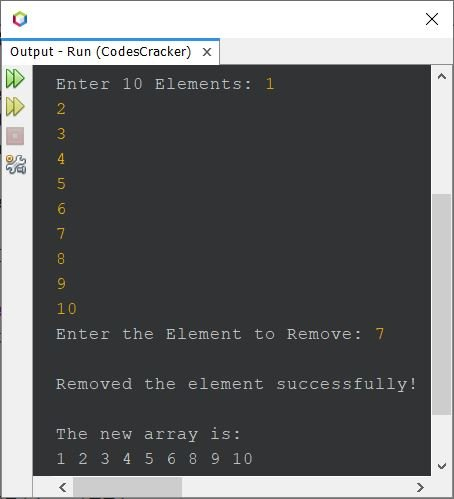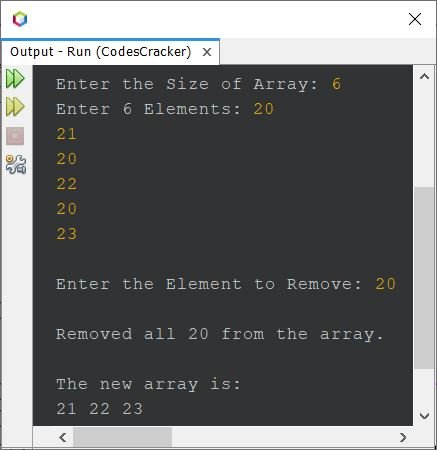# Java Program to Remove an Element from an Array

## Remove Element from an Array in Java - Basic Version

The question is, write a Java program to remove a given element from a given array. The program given below is its answer. This program shifts elements to one index back, from the index, where the element gets found.

```import java.util.Scanner;

public class CodesCracker
{
public static void main(String[] args)
{
int i, j, size=10, element;
int[] arr = new int[size];
Scanner scan = new Scanner(System.in);

System.out.print("Enter 10 Elements: ");
for(i=0; i<size; i++)
arr[i] = scan.nextInt();

System.out.print("Enter the Element to Remove: ");
element = scan.nextInt();

for(i=0; i<size; i++)
{
if(element==arr[i])
{
for(j=i; j<(size-1); j++)
arr[j] = arr[j+1];
System.out.println("\nRemoved the element successfully!");
break;
}
}

System.out.println("\nThe new array is: ");
for(i=0; i<(size-1); i++)
System.out.print(arr[i]+ " ");
}
}```

The snapshot given below shows the sample run of above program with user input 1, 2, 3, 4, 5, 6, 7, 8, 9, 10 as ten elements and 7 as element to delete or remove:## Remove Element from Array in Java - Complete Version

Since previous program does not allows user to define the size of array. Also, that program is not correct, when user enters an element that does not available in the array, or an element that occurs multiple times. Therefore, I've modified that program, and created a new one. Here is the modified version of previous program:

```import java.util.Scanner;

public class CodesCracker
{
public static void main(String[] args)
{
int i, j, size, element, count=0;
Scanner scan = new Scanner(System.in);

System.out.print("Enter the Size of Array: ");
size = scan.nextInt();
int[] arr = new int[size];

System.out.print("Enter " +size+ " Elements: ");
for(i=0; i<size; i++)
arr[i] = scan.nextInt();

System.out.print("\nEnter the Element to Remove: ");
element = scan.nextInt();

for(i=0; i<size; i++)
{
if(element==arr[i])
{
for(j=i; j<(size-1); j++)
arr[j] = arr[j+1];
size--;
i--;
count++;
}
}

if(count==0)
else if(count==1)
{
System.out.println("\nRemoved the element successfully!");
System.out.println("\nThe new array is: ");
for(i=0; i<size; i++)
System.out.print(arr[i]+ " ");
}
else
{
System.out.println("\nRemoved all " +element+ " from the array.");
System.out.println("\nThe new array is: ");
for(i=0; i<size; i++)
System.out.print(arr[i]+ " ");
}
}
}```

Here is its sample run with user input 6 as size, 20, 21, 20, 22, 20, 23 as six elements, and 20 as element to delete:In above program, the following block of for loop:

```for(i=0; i<size; i++)
{
if(element==arr[i])
{
for(j=i; j<(size-1); j++)
arr[j] = arr[j+1];
size--;
i--;
count++;
}
}```

can also be written using while loop, as shown below:

```i=0;
while(i<size)
{
if(element==arr[i])
{
j=i;
while(j<(size-1))
{
arr[j] = arr[j+1];
j++;
}
size--;
i--;
count++;
}
i++;
}```

#### Same Program in Other Languages

Java Online Test

« Previous Program Next Program »